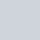# Calculating the U-value of walls in Revit

What is the U-Value?  It is:
Also known as the U-factor or coefficient of heat transmission, a measure of the rate of non-solar heat loss or gain through a material or assembly. U-values gauge how well a material allows heat to pass through.
http://www.daviddarling.info/encyclopedia/U/AE_U-value.html

autodesk.de recently carried a detailed post on formulas related to the U-value.

### Summary

To get the U value of a wall structure, add the sum of the heat transfer coefficients for the wall types specified thermal resistance R T by this value to be used as a divisor.

With walls so:

U = 1 / (Rt (from Revit) 0.17 (K · m) / W)### Leave a ReplySubscribe
Notify of